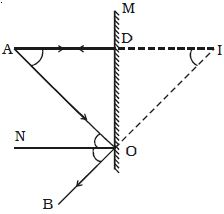# Describe Image in a Plane Mirror

Image in a Plane Mirror: Let consider a point object A placed in front of a plane mirror M as shown in the Figure. Consider a ray of light AO from the point object incident on the mirror and reflected along OB. Draw the normal ON to the mirror at O.The angle of incidence AON = angle of reflection BON

Another ray AD incident normally on the mirror at D is reflected back along DA. When BO and AD are produced backwards, they meet at I. Thus the rays reflected from M appear to come from a point I behind the mirror. From the figure;

Angle AON = Angle DAO, alternate angles and Angle BON = Angle DIO, corresponding angles it follows that Angle DAO = Angle DIO.

The triangles ODA and ODI are congruent.